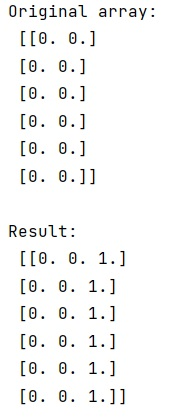# Python | Add Column to NumPy 2D Array

In this tutorial, we will learn how to add column to NumPy 2d (Two-dimensional) array in Python? By Pranit Sharma Last updated : April 17, 2023

## Overview

Suppose that we are given a 2D NumPy array and we need to add a column in this array.

Note that, we have a 2D array with multiple rows and multiple columns and we have another column-like array with multiple rows.

## How to Add Column to NumPy 2D Array?

To add a column to NumPy 2D array, just add a column with multiple rows using the `numpy.hstack()` method which adds a column horizontally in the original 2D array.

Let us understand with the help of an example,

## Python Program to Add Column to NumPy 2D Array

```# Import numpy
import numpy as np

# Creating an array
arr = np.zeros((6,2))

# Display original array
print("Original array:\n",arr,"\n")

# Creating single column array
col = np.ones((6,1))

res = np.hstack((arr,col))

# Display result
print("Result:\n",res)
```

### Output